Function Repository Resource:

# HampelFilter

Remove outliers from a signal using a Hampel window

Contributed by: Jon McLoone
 ResourceFunction["HampelFilter"][data] removes outliers from data. ResourceFunction["HampelFilter"][data,w] uses a moving window of size w. ResourceFunction["HampelFilter"][data,w,d] removes outliers that are more than d standard deviations away from the expectation.

## Details

ResourceFunction["HampelFilter"] replaces outliers with the median of a moving window. By default, three points on each side are used.
A point is considered an outlier if its value is more than one standard deviation from the moving median.
The distance from the moving median is estimated using MeanDeviation.

## Examples

### Basic Examples (2)

Signal data with outliers:

 In:=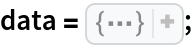In:=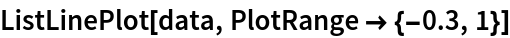Out=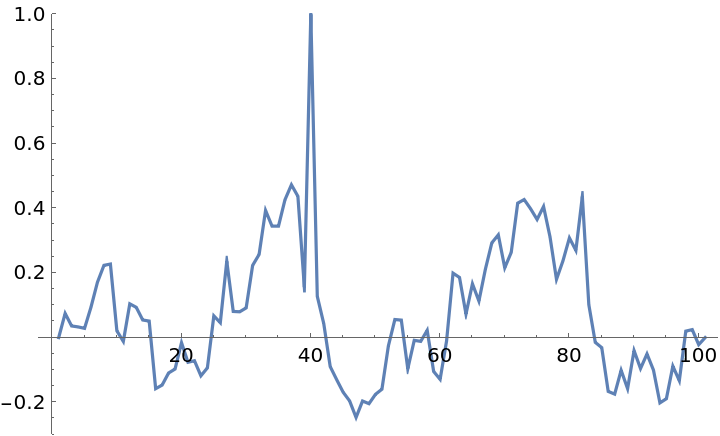Remove outliers from the data:

 In:=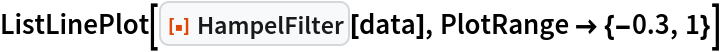Out=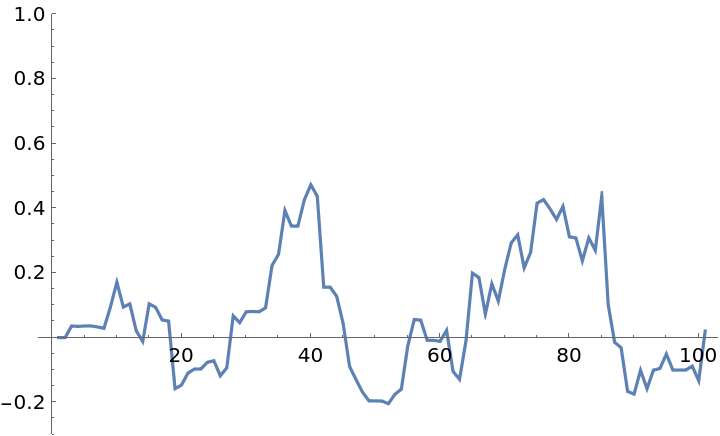### Scope (4)

Reduce the window size used to decide if a point is an outlier:

 In:=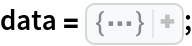In:=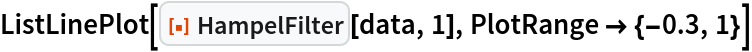Out=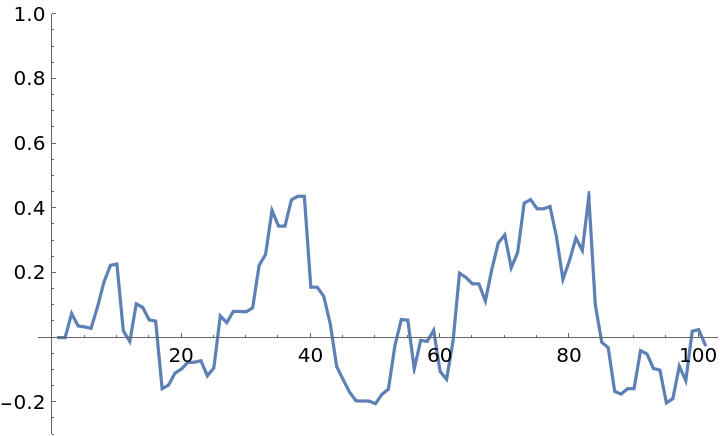The window size can be also be increased:

 In:=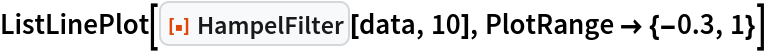Out=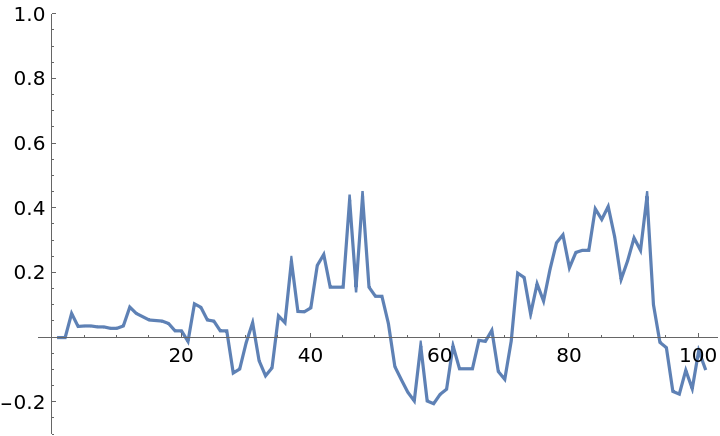Reduce the outlier threshold to make HampelFilter more sensitive:

 In:=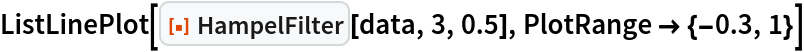Out=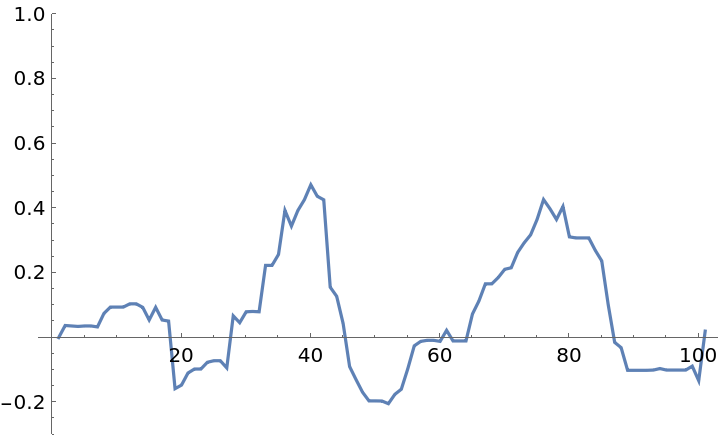Increasing the threshold will adjust fewer points:

 In:=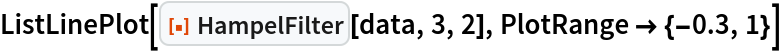Out=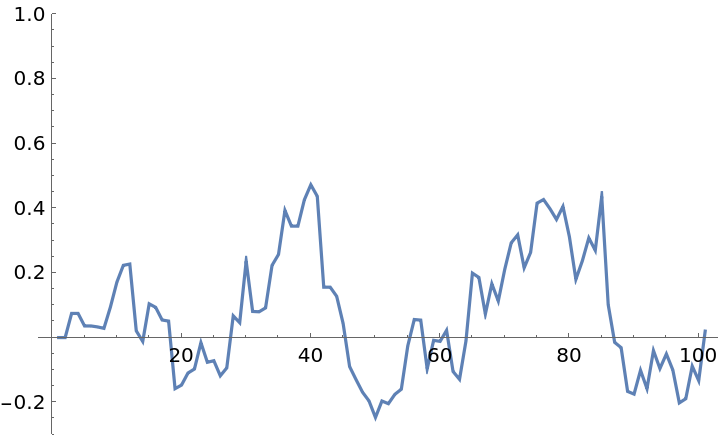Jon McLoone

## Version History

• 1.0.0 – 22 March 2021